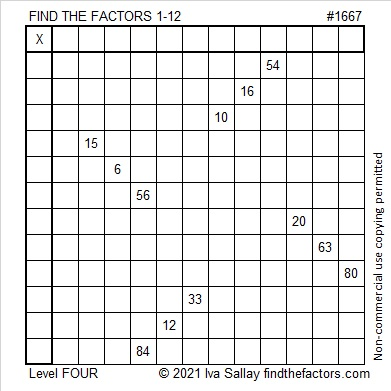# 1667 and Level 4

Contents

### Today’s Puzzle:

Use logic to write the numbers 1 to 12 in both the first column and the top row so that those numbers and the given clues function like a multiplication table.### Factors of 1667:

• 1667 is a prime number.
• Prime factorization: 1667 is prime.
• 1667 has no exponents greater than 1 in its prime factorization, so √1667 cannot be simplified.
• The exponent in the prime factorization is 1. Adding one to that exponent we get (1 + 1) = 2. Therefore 1667 has exactly 2 factors.
• The factors of 1667 are outlined with their factor pair partners in the graphic below.How do we know that 1667 is a prime number? If 1667 were not a prime number, then it would be divisible by at least one prime number less than or equal to √1667. Since 1667 cannot be divided evenly by 2, 3, 5, 7, 11, 13, 17, 19, 23, 29, 31, or 37, we know that 1667 is a prime number.

### More About the Number 1667:

Look at these consecutive number facts about the number 1667:
833 + 834 = 1667.
834² – 833² = 1667.

As the chart below shows, 1667 was ALMOST the fourth consecutive prime number ending in 7. Too bad prime number 1663 got in the way of that happening.This site uses Akismet to reduce spam. Learn how your comment data is processed.